### PROC LP Statement

PROC LP options ;

This statement invokes the procedure. The following options can appear in the PROC LP statement.

#### Data Set Options

ACTIVEIN=SAS-data-set

names the SAS data set containing the active nodes in a branch-and-bound tree that is to be used to restart an integer program.

ACTIVEOUT=SAS-data-set

names the SAS data set in which to save the current branch-and-bound tree of active nodes.

DATA=SAS-data-set

names the SAS data set containing the problem data. If the DATA= option is not specified, PROC LP uses the most recently created SAS data set.

DUALOUT=SAS-data-set

names the SAS data set that contains the current dual solution (shadow prices) on termination of PROC LP. This data set contains the current dual solution only if PROC LP terminates successfully.

MPSOUT=SAS-data-set

names the SAS data set that contains converted sparse or dense format input data in MPS format. Invoking this option directs the LP procedure to halt before attempting optimization. For more information about the MPS-format SAS data set, see Chapter 15: The MPS-Format SAS Data Set in SAS/OR 12.1 User's Guide: Mathematical Programming.

PRIMALIN=SAS-data-set

names the SAS data set that contains a feasible solution to the problem defined by the DATA= data set. The data set specified in the PRIMALIN= option should have the same format as a data set saved using the PRIMALOUT= option. Specifying the PRIMALIN= option is particularly useful for continuing iteration on a problem previously attempted. It is also useful for performing sensitivity analysis on a previously solved problem.

PRIMALOUT=SAS-data-set

names the SAS data set that contains the current primal solution when PROC LP terminates.

SPARSEDATA

tells PROC LP that the data are in the sparse input format. If this option is not specified, PROC LP assumes that the data are in the dense input format. See the section Sparse Data Input Format for information about the sparse input format.

TABLEAUOUT=SAS-data-set

names the SAS data set in which to save the final tableau.

#### Display Control Options

FLOW

requests that a journal of pivot information (the Iteration Log) be displayed after every PRINTFREQ= iterations. This includes the names of the variables entering and leaving the basis, the reduced cost of the entering variable, and the current objective value.

FUZZ=e

displays all numbers within e of zero as zeros. The default value is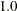E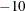.

NOFLOW

is the inverse of the FLOW option.

NOPARAPRINT

is the inverse of the PARAPRINT option.

NOPRINT

suppresses the display of the Variable, Constraint, and Sensitivity Analysis summaries. This option is equivalent to the PRINTLEVEL=0 option.

NOTABLEAUPRINT

is the inverse of the TABLEAUPRINT option.

PARAPRINT

indicates that the solution be displayed at each pivot when performing parametric programming.

PRINT

is the inverse of the NOPRINT option.

PRINTFREQ=m

indicates that after every mth iteration, a line in the (Integer) Iteration Log be displayed. The default value is 1.

PRINTLEVEL=i

indicates the amount of displaying that the procedure should perform.

PRINTLEVEL=-2

only messages to the SAS log are displayed

PRINTLEVEL=-1

is equivalent to NOPRINT unless the problem is infeasible. If it is infeasible, the infeasible rows are displayed in the Constraint Summary along with the Infeasible Information Summary.

PRINTLEVEL=0

is identical to NOPRINT

PRINTLEVEL=1

all output is displayed

The default value is 1.

TABLEAUPRINT

indicates that the final tableau be displayed.

#### Interactive Control Options

ENDPAUSE

requests that PROC LP pause before displaying the solution. When this pause occurs, you can enter the RESET, SHOW, PRINT, RUN, and QUIT statements.

FEASIBLEPAUSE

requests that PROC LP pause after a feasible (not necessarily integer feasible) solution has been found. At a pause, you can enter the RESET, SHOW, PRINT, PIVOT, RUN, and QUIT statements.

IFEASIBLEPAUSE=n

requests that PROC LP pause after every n integer feasible solutions. At a pause, you can enter the RESET, SHOW, PRINT, IPIVOT, PIVOT, RUN, and QUIT statements. The default value is 99999999.

IPAUSE=n

requests that PROC LP pause after every n integer iterations. At a pause, you can enter RESET, SHOW, PRINT, IPIVOT, PIVOT, RUN, and QUIT statements. The default value is 99999999.

NOENDPAUSE

is the inverse of the ENDPAUSE option.

NOFEASIBLEPAUSE

is the inverse of the FEASIBLEPAUSE option.

PAUSE=n

requests that PROC LP pause after every n iterations. At a pause, you can enter the RESET, SHOW, PRINT, IPIVOT, PIVOT, RUN, and QUIT statements. The default value is 99999999.

PROXIMITYPAUSE=r

causes the procedure to pause if at least one integer feasible solution has been found and the objective value of the current best integer solution can be determined to be within r units of the optimal integer solution. This distance, called proximity, is also displayed on the Integer Iteration Log. Note that the proximity is calculated using the minimum (maximum if the problem is maximization) objective value among the nodes that remain to be explored in the branch-and-bound tree as a bound on the value of the optimal integer solution. Following the first PROXIMITYPAUSE= pause, in order to avoid a pause at every iteration thereafter, it is recommended that you reduce this measure through the use of a RESET statement. Otherwise, if any other option or statement that causes the procedure to pause is used while the PROXIMITYPAUSE= option is in effect, pause interferences may occur. When this pause occurs, you can enter the RESET, SHOW, PRINT, IPIVOT, PIVOT, RUN, and QUIT statements. The default value is 0.

requests that PROC LP pause after the data have been read and the initial basis inverted. When this pause occurs, you can enter the RESET, SHOW, PRINT, IPIVOT, PIVOT, RUN, and QUIT statements.

#### Preprocessing Control Options

NOPREPROCESS

is the inverse of the PREPROCESS option.

PEPSILON=e

specifies a positive number close to zero. This value is an error tolerance in the preprocessing. If the value is too small, any marginal changes may cause the preprocessing to repeat itself. However, if the value is too large, it may alter the optimal solution or falsely claim that the problem is infeasible. The default value isE
.

PMAXIT=n

performs at most n preprocessings. Preprocessing repeats itself if it improves some bounds or fixes some variables. However when a problem is large and dense, each preprocessing may take a significant amount of CPU time. This option limits the number of preprocessings PROC LP performs. It can also reduce the build-up of round-off errors. The default value is 100.

PREPROCESS

performs preprocessing techniques. See the section Preprocessing for further discussion.

#### Branch-and-Bound Algorithm Control Options

AUTO, AUTO(m,n)

automatically sets and adjusts the value of the CONTROL= option. Initially, it sets CONTROL=0.70, concentrating on finding an integer feasible solution or an upper bound. When an upper bound is found, it sets CONTROL=0.5, concentrating on efficiency and lower bound improvement. When the number of active problems exceeds m, it starts to gradually increase the value of the CONTROL= option to keep the size of active problems under control. When total active problems exceed n, CONTROL=1 will keep the active problems from growing further. You can alter the automatic process by resetting the value of the CONTROL= option interactively.

The default values of m and n are 20000 and 250000, respectively. You can change the two values according to your computer’s space and memory capacities.

BACKTRACK=rule

specifies the rule used to choose the next active problem when backtracking is required. One of the following can be specified:

• BACKTRACK=LIFO

• BACKTRACK=FIFO

• BACKTRACK=OBJ

• BACKTRACK=PROJECT

• BACKTRACK=PSEUDOC

• BACKTRACK=ERROR

The default value is OBJ. See the section Integer Programming for further discussion.

BINFST

requests that PROC LP branch on binary variables first when integer and binary variables are present. The reasoning behind this is that a subproblem will usually be fathomed or found integer feasible after less than 20% of its variables have been fixed. Considering binary variables first attempts to reduce the size of the branch-and-bound tree. It is a heuristic technique.

CANSELECT=rule

specifies the rule used to choose the next active problem when backtracking is not required or used. One of the following can be specified:

• CANSELECT=LIFO

• CANSELECT=FIFO

• CANSELECT=OBJ

• CANSELECT=PROJECT

• CANSELECT=PSEUDOC

• CANSELECT=ERROR

The default value is LIFO. See the section Integer Programming for further discussion.

CONTROL=r

specifies a number between 0 and 1. This option combines CANSELECT= and other rules to choose the next active problem. It takes into consideration three factors: efficiency, improving lower bounds, and improving upper bounds. When r is close to 0, PROC LP concentrates on improving lower bounds (upper bounds for maximization). However, the efficiency per integer iteration is usually the worst. When r is close to 1, PROC LP concentrates on improving upper bounds (lower bounds for maximization). In addition, the growth of active problems will be controlled and stopped at r = 1. When its value is around 0.5, PROC LP will be in the most efficient state in terms of CPU time and integer number of iterations. The CONTROL= option will be automatically adjusted when the AUTO option is applied.

DELTAIT=r

is used to modify the exploration of the branch-and-bound tree. If more than r integer iterations have occurred since the last integer solution was found, then the procedure uses the backtrack strategy in choosing the next node to be explored. The default value is 3 times the number of integer variables.

DOBJECTIVE=r

specifies that PROC LP should discard active nodes that cannot lead to an integer solution with the objective at least as small (or as large for maximizations) as the objective of the relaxed problem plus (minus) r. The default value is.

IEPSILON=e

requests that PROC LP consider an integer variable as having an integer value if its value is within e units of an integer. The default value isE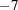.

IMAXIT=n

performs at most n integer iterations. The default value is 100.

IOBJECTIVE=r

specifies that PROC LP should discard active nodes unless the node could lead to an integer solution with the objective smaller (or larger for maximizations) than r. The default value isfor minimization (for maximization).

LIFOTYPE=c

specifies the order in which to add the two newly branched active nodes to the LIFO list.

LIFOTYPE=0

add the node with minimum penalty first

LIFOTYPE=1

add the node with maximum penalty first

LIFOTYPE=2

add the node resulting from adding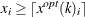first

LIFOTYPE=3

add the node resulting from adding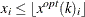first

The default value is 0.

NOAUTO

is the inverse of the AUTO option.

NOBINFST

is the inverse of the BINFST option.

NOPOSTPROCESS

is the inverse of the POSTPROCESS option.

PENALTYDEPTH=m

requests that PROC LP examine m variables as branching candidates when VARSELECT=PENALTY. If the PENALTYDEPTH= option is not specified when VARSELECT=PENALTY, then all of the variables are considered branching candidates. The default value is the number of integer variables. See the section Integer Programming for further discussion.

POBJECTIVE=r

specifies that PROC LP should discard active nodes that cannot lead to an integer solution with objective at least as small as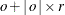(at least as large as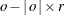for maximizations) whereis the objective of the relaxed noninteger constrained problem. The default value is.

POSTPROCESS

attempts to fix binary variables globally based on the relationships among the reduced cost and objective value of the relaxed problem and the objective value of the current best integer feasible solution.

PWOBJECTIVE=r

specifies a percentage for use in the automatic update of the WOBJECTIVE= option. If the WOBJECTIVE= option is not specified in PROC LP, then when an integer feasible solution is found, the value of the option is updated to be b + qr where b is the best bound on the value of the optimal integer solution and q is the current proximity. Note that for maximizations, b - qr is used. The default value is 0.95.

TREETYPE=i

specifies a data compression algorithm.

TREETYPE=0

no data compression

TREETYPE=1

Huffman coding compression routines

TREETYPE=2

TREETYPE=3

For IP or MIP problems, the basis and bounds information of each active node is saved to a utility file. When the number of active nodes increases, the size of the utility file becomes larger and larger. If PROC LP runs into a disk problem, like disk full … or writing failure …, you can use this option to compress the utility file. For more information on the data compression routines, refer to Nelson (1992). The default value is 0.

VARSELECT=rule

specifies the rule used to choose the branching variable on an integer iteration.

• VARSELECT=CLOSE

• VARSELECT=PRIOR

• VARSELECT=PSEUDOC

• VARSELECT=FAR

• VARSELECT=PRICE

• VARSELECT=PENALTY

The default value is FAR. See the section Integer Programming for further discussion.

WOBJECTIVE=r

specifies that PROC LP should delay examination of active nodes that cannot lead to an integer solution with objective at least as small (as large for maximizations) as r, until all other active nodes have been explored. The default value isfor minimization (for maximization).

#### Sensitivity/Parametric/Ranging Control Options

NORANGEPRICE

is the inverse of the RANGEPRICE option.

NORANGERHS

is the inverse of the RANGERHS option.

PRICEPHI=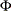specifies the limit for parametric programming when perturbing the price vector. See the section Parametric Programming for further discussion. See Example 6.5 for an illustration of this option.

RANGEPRICE

indicates that range analysis is to be performed on the price coefficients. See the section Range Analysis for further discussion.

RANGERHS

indicates that range analysis is to be performed on the right-hand-side vector. See the section Range Analysis for further discussion.

RHSPHI=specifies the limit for parametric programming when perturbing the right-hand-side vector. See the section Parametric Programming for further discussion.

#### Simplex Algorithm Control Options

DEVEX

indicates that the devex method of weighting the reduced costs be used in pricing (Harris 1975).

EPSILON=e

specifies a positive number close to zero. It is used in the following instances:

During phase 1, if the sum of the basic artificial variables is within e of zero, the current solution is considered feasible. If this sum is not exactly zero, then there are artificial variables within e of zero in the current solution. In this case, a note is displayed on the SAS log.

During phase 1, if all reduced costs are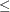e for nonbasic variables at their lower bounds and
e for nonbasic variables at their upper bounds and the sum of infeasibilities is greater than e, then the problem is considered infeasible. If the maximum reduced cost is within e of zero, a note is displayed on the SAS log.

During phase 2, if all reduced costs aree for nonbasic variables at their lower bounds ande for nonbasic variables at their upper bounds, then the current solution is considered optimal.

During phases 1, 2, and 3, the EPSILON= option is also used to test if the denominator is different from zero before performing the ratio test to determine which basic variable should leave the basis.

The default value isE.

GOALPROGRAM

specifies that multiple objectives in the input data set are to be treated as sequential objectives in a goal-programming model. The value of the right-hand-side variable in the objective row gives the priority of the objective. Lower numbers have higher priority.

INFINITY=r

specifies the largest number PROC LP uses in computation. The INFINITY= option is used to determine when a problem has an unbounded variable value. The default value is the largest double precision number. 

INVFREQ=m

reinverts the current basis matrix after m major and minor iterations. The default value is 100.

INVTOL=r

reinverts the current basis matrix if the largest element in absolute value in the decomposed basis matrix is greater than r. If after reinversion this condition still holds, then the value of the INVTOL= option is increased by a factor of 10 and a note indicating this modification is displayed on the SAS log. When r is frequently exceeded, this may be an indication of a numerically unstable problem. The default value is 1000.

MAXIT=n

simultaneously sets the values of the MAXIT1=, MAXIT2=, MAXIT3=, and IMAXIT= options.

MAXIT1=n

performs at most nphase 1 iterations. The default value is 100.

MAXIT2=n

performs at most nphase 2 iterations. If MAXIT2=0, then only phase 1 is entered so that on successful termination PROC LP will have found a feasible, but not necessarily optimal, solution. The default value is 100.

MAXIT3=n

performs at most nphase 3 iterations. All dual pivots are counted as phase 3 pivots. The default value is 99999999.

NODEVEX

is the inverse of the DEVEX option.

PARARESTORE

indicates that following a parametric programming analysis, PROC LP should restore the basis.

PHASEMIX=r

specifies a number between 0 and 1. When the number is positive, PROC LP tries to improve the objective function of phase 2 during phase 1. The PHASEMIX= option is a weight factor of the phase 2 objective function in phase 1. The default value is 0.

PRICE=m

specifies the number of columns to subset when multiple pricing is used in selecting the column to enter the basis (Greenberg 1978). The type of suboptimization used is determined by the PRICETYPE= option. See the section Pricing for a description of this process.

PRICETYPE=pricetype

specifies the type of multiple pricing to be performed. If this option is specified and the PRICE= option is not specified, then PRICE= is assumed to be 10. Valid values for the PRICETYPE= option are

• PRICETYPE=COMPLETE

• PRICETYPE=DYNAMIC

• PRICETYPE=NONE

• PRICETYPE=PARTIAL

The default value is PARTIAL. See the section Pricing for a description of this process.

RANDOMPRICEMULT=r

specifies a number between 0 and 1. This option sets a limit, in phase 1, on the number of iterations when PROC LP will randomly pick the entering variables. The limit equals r times the number of nonbasic variables, or the number of basic variables, whichever is smaller. The default value of the RANDOMPRICEMULT= option is 0.01.

REPSILON=e

specifies a positive number close to zero. The REPSILON= option is used in the ratio test to determine which basic variable is to leave the basis. The default value isE.

SCALE=scale

specifies the type of scaling to be used. Valid values for the SCALE= option are

• SCALE=BOTH

• SCALE=COLUMN

• SCALE=NONE

• SCALE=ROW

The default value is BOTH. See the section Scaling for further discussion.

SMALL=e

specifies a positive number close to zero. Any element in a matrix with a value less than e is set to zero. The default value is machine dependent.

TIME=t

checks at each iteration to see if t seconds have elapsed since PROC LP began. If more than t seconds have elapsed, the procedure pauses and displays the current solution. The default value is 120 seconds.

U=r

enables PROC LP to control the choice of pivots during LU decomposition and updating the basis matrix. The variable r should take values between EPSILON and 1.0 because small values of r bias the algorithm toward maintaining sparsity at the expense of numerical stability and vice versa. The more sparse the decomposed basis is, the less time each iteration takes. The default value is 0.1.

 This value is system dependent.